# NCERT Solutions for Class 8 Maths Chapter 11 Mensuration

NCERT Solutions for Class 8 Maths Chapter 11 Mensuration Exercise 11.1

Ex 11.1 Class 8 Maths Question 1.
A square and a rectangular field with measurements as given in the figure have the same perimeter. Which field has a larger area?
Solution:
Perimeter of figure (a) = 4 × side = 4 × 60 = 240 mPerimeter of figure (b) = 2 [l + b]
Perimeter of figure (b) = Perimeter of figure (a)
2[l + b] = 240
⇒ 2 [80 + b] = 240
⇒ 80 + b = 120
⇒ b = 120 – 80 = 40 m
Area of figure (a) = (side)2 = 60 × 60 = 3600 m2
Area of figure (b) = l × b = 80 × 40 = 3200 m2
So, area of figure (a) is longer than the area of figure (b).

Ex 11.1 Class 8 Maths Question 2.
Mrs Kaushik has a square plot with the measurement as shown in the figure. She wants to construct a house in the middle of the plot. A garden is developed around the house. Find the total cost of developing a garden around the house at the rate of ₹ 55 per m2.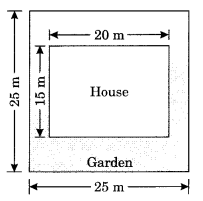Solution:
Area of the plot = side × side = 25 m × 25 m = 625 m2
Area of the house = l × b = 20 m × 15 m = 300 m2
Area of the garden to be developed = Area of the plot – Area of the house = 625 m2 – 300 m2 = 325 m2
Cost of developing the garden = ₹ 325 × 55 = ₹ 17875

Ex 11.1 Class 8 Maths Question 3.
The shape of a garden is rectangular in the middle and semicircular at the ends as shown in the diagram. Find the area and the perimeter of this garden. [Length of rectangle is 20 – (3.5 + 3.5) metres]Solution:
Length of the rectangle = 20 – (3.5 + 3.5) = 20 – 7 = 13 m
Area of the rectangle = l × b = 13 × 7 = 91 m2
Area of two circular ends = 2($$\frac { 1 }{ 2 }$$ πr2)
= πr2
= $$\frac { 22 }{ 7 }$$ × $$\frac { 7 }{ 2 }$$ × $$\frac { 7 }{ 2 }$$
= $$\frac { 77 }{ 2 }$$ m2
= 38.5 m2
Total area = Area of the rectangle + Area of two ends = 91 m2 + 38.5 m2 = 129.5 m2
Total perimeter = Perimeter of the rectangle + Perimeter of two ends
= 2 (l + b) + 2 × (πr) – 2(2r)
= 2 (13 + 7) + 2($$\frac { 22 }{ 7 }$$ × $$\frac { 7 }{ 2 }$$) – 4 × $$\frac { 7 }{ 2 }$$
= 2 × 20 + 22 – 14
= 40 + 22 – 14
= 48 m

Ex 11.1 Class 8 Maths Question 4.
A flooring tile has the shape of a parallelogram whose base is 24 cm and the corresponding height is 10 cm. How many such tiles are required to cover a floor of area 1080 m2? (If required you can split the tiles in whatever way you want to fill up the corners).
Solution:
Area of the floor = 1080 m2 = 1080 × 10000 cm2 = 10800000 cm2 [∵ 1 m2 = 10000 cm2]
Area of 1 tile = 1 × base × height = 1 × 24 × 10 = 240 cm2
Number of tiles required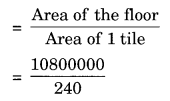= 45000 tiles

Ex 11.1 Class 8 Maths Question 5.
An ant is moving around a few food pieces of different shapes scattered on the floor. For which food-piece would the ant have to take a longer round? Remember, the circumference of a circle can be obtained by using the expression C = 2πr, where r is the radius of the circle.Solution: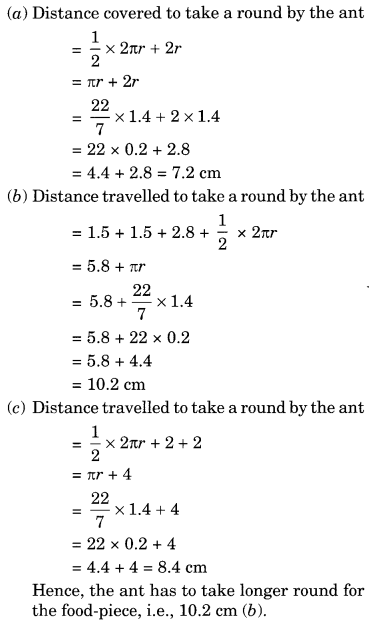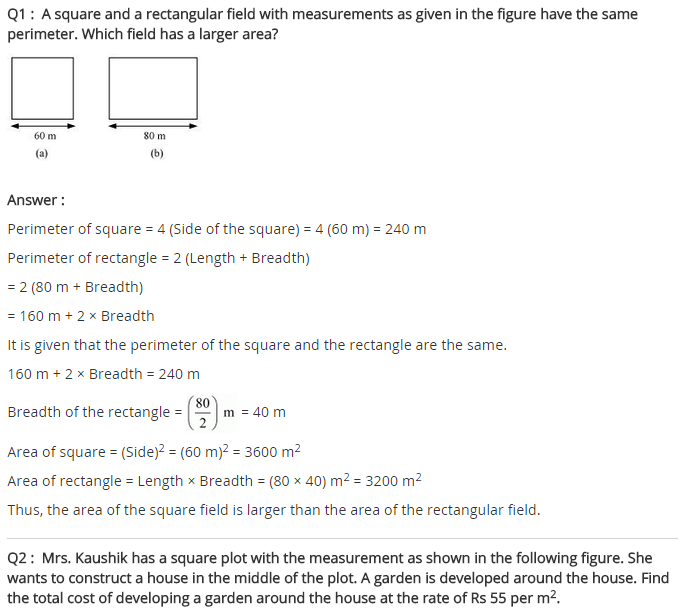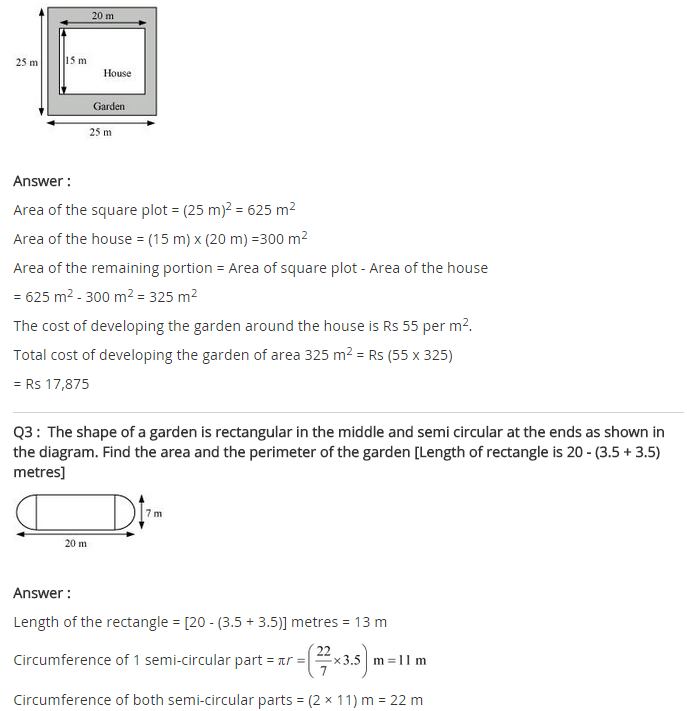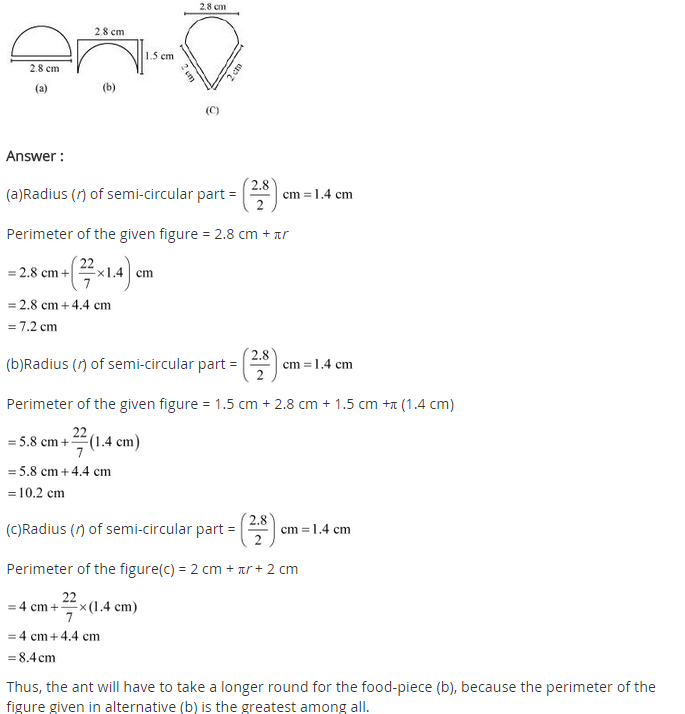+